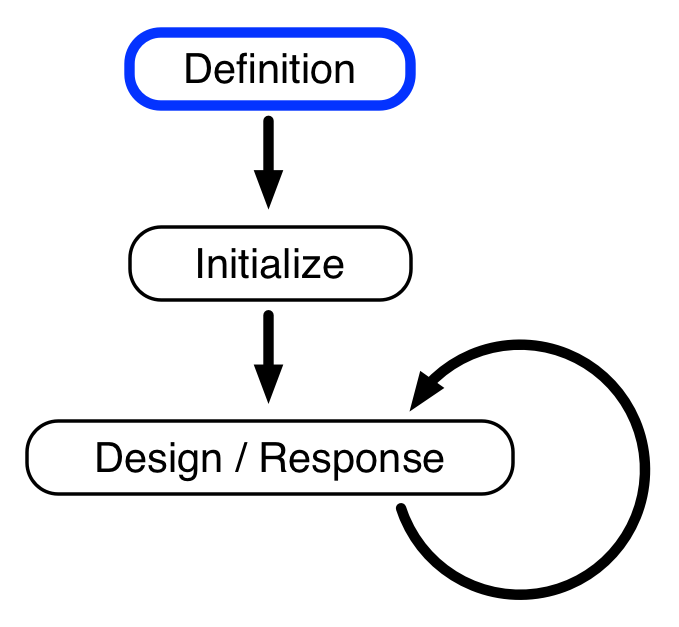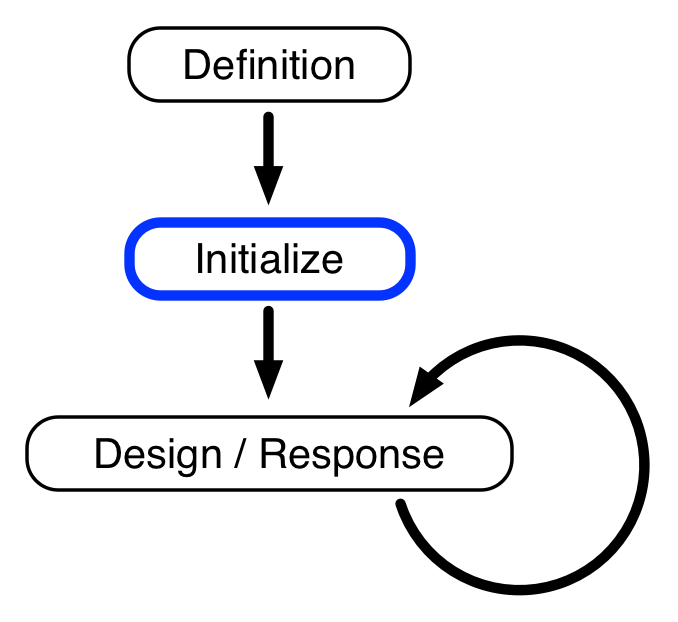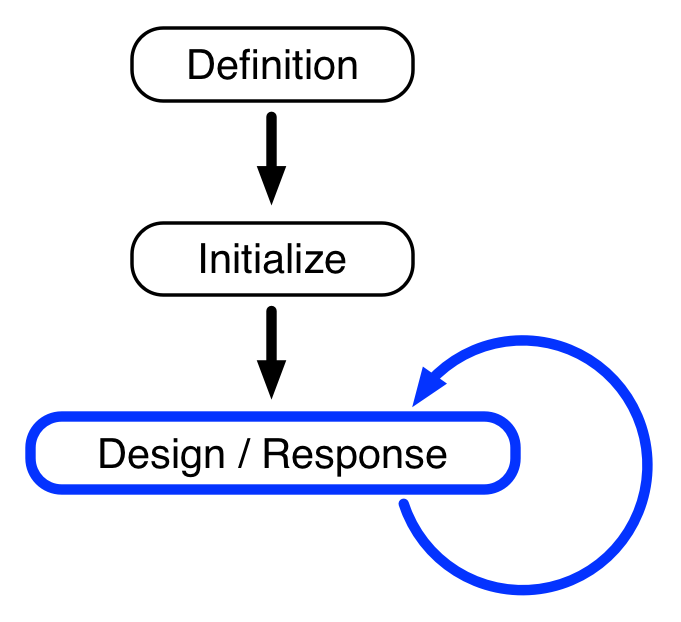## daptics Overview

You are here because you want to optimize a complex experiment. You have some way of judging the quality of your experiments, by measuring an experimental response. Your experiment is complex, because it has several experimental parameters (e.g., between 4 and 20) that interact with each other in unknown, unpredictable ways. You want to optimize these parameters, i.e., find values of the experimental parameters that lead to desired experimental responses. You will have some way of executing experiments in an automated or semi-automated way (e.g. using a high-throughput experimental platform).

We will consider your experiments as being grouped into generations. daptics is a tool for designing experiments for each generation, based on sophisticated machine-learning modeling of data from all past generations, with the aim of optimizing experimental responses.

Your experiments are expensive, so that each generation typically won't contain more than 10's, 100's, or few 1000's of experiments. Prediction of the next generation of experiments is therefore a small data problem. Building predictive models using machine-learning techniques for small data problems requires special methods (in contrast to methods used for big data), made conveniently available to you with the daptics web interface.

Your use of daptics entails three basic phases, two for initialization, and one that is repeated over and over with each generation:

### 1. Experimental space definitiondaptics algorithms begin with the Experimental Space Definition (ESD), which is a definition of all possible experiments to be explored. It is a space of discrete experimental possibilities, specified with a discrete set of possible values for each experimental variable.

You start by choosing the type of experimental space in which your experiments are defined. Currently there are two options:

Factorial, where you specify the experimental space as a list of experimental parameters (e.g. concentrations, temperatures, etc), and of the discrete values each parameter may take when you execute an experiment. The experimental space is given by all possible combinations of values, one for each parameter. Specification of each variable includes the variable's type; for factorial experiments, the variable may be either numerical or categorical.

Mixture, where you specify the experimental space as a list of experimental parameters, and a range of integer values that each parameter may take. These values correspond to the number of units of that parameter in an experiment (for example, the number of drops of solution in an experiment set up by a liquid handling robot). The number of units, summed over all parameters, is equal to a constant usum for all experiments. That implies that if an experiment contains u units of a certain parameter, the ratio u/usum represents the proportion of that parameter in the mixture. For factorial experiments the variables must all be of type unit.

After choosing the type of experimental space, and specifying experimental parameters and values, you then specify the generation parameters, Np = population size and Nr = number of replicates. These two numbers determine how many experiments there will be in a generation: Nexp = Np (Nr + 1).

• Price is determined by the ESD and population size, according to our dynamical pricing algorithm (lower-complexity experiments are cheaper, higher-complexity experiments are more expensive).

• for low-complexity experiments: daptics has a free zone. The free zone is defined for factorial experiments having up to 4 numeric parmeters, each with up to 8 values.

• for high-complexity experiments: our web interface to daptics hits a maximal experimental complexity when ESDs reach 20 parameters with 20 values each, and when the population size reaches 2500 experiments per generation. daptics can be configured to handle larger ESDs and population sizes; please contact our sales team to discuss pricing for such special projects.

### 2. Initial experimentsBefore launching a daptics campaign, you may want to enter experimental results (experiments and response measurements) that you have gathered in preliminary studies, e.g., calibration runs, as initial experiments. daptics may be initialized with the results of these initial experiments. This initialization is, however completely optional; daptics may proceed without them.

To be usable by daptics, initial experiments must lie in an expanded variant of the experimental space determined in the previous experimental space definition phase. This means that each experiment must have a specified value for each of the parameters in the experimental space definition (and, if the space is a mixture, respect the constraint on the total number of units), but it is not necessary that its parameter values correspond to particular values specified in the experimental space definition.

### 3. Experiment design and response measurementsdaptics iteratively chooses designs of Nexp experiments, each selected from your experimental space, called generations. The first generation is typically exploratory, aimed at "covering" the experimental space, while keeping into account any initial experiments you may have entered in the previous phase. At the end of every generation, daptics will: (a) use all available experimental results to build a model that predicts which, among all untried experiments, are those most likely to have good experimental response; (b) identify the regions of the experimental space that have not been yet covered by the tried experiments; (c) choose the design for the following generation based on (a) and (b).

In addition to the experiments chosen by daptics for the current generation, you may optionally perform extra (additional) experiments, either to explore your own intuition, or to further validate results from previous generations, e.g., for calibration reasons. daptics will use results from extra experiments the same way it does with results from daptics-designed experiments.